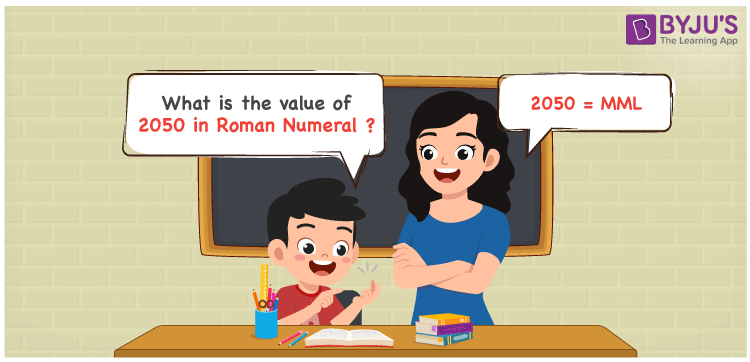# 2050 in Roman Numerals

2050 in Roman Numerals is MML. Any Roman numeral uses only the 7 primary symbols which are I, V, X, L, C, D, and M, Where M represents 1000, X represents 10 and L represents 50. What are Roman numerals? can be referred for more details. The details on how to write 2050 are given in the next section of this article.

 Number Roman Numeral 2050 MML

## How to Write 2050 in Roman Numerals?To convert 2050 in Roman Numerals, we need to represent 2050 as the sum of the fundamental symbols. 2050 can be broken down as the sum of 1000, 1000 and 50.

All these 3 numbers have fundamental symbols, and hence 2050 is expressed as MML.

Let us understand it numerically;

2050 = 1000 + 1000 + 50

2050 = M + M + L

2050 = MML.

## Video Lesson on Roman Numerals## Frequently Asked Questions on 2050 in Roman Numerals

Q1

### How to write the number 2050 in Roman Numerals?

2050 in Roman Numerals is written as MML.
Q2

### Do MML and DDML represent Roman Numerals?

No. MML represents Roman Numerals and it is the number 2050, whereas DDML is an invalid Roman numeral.
Q3

### How to express the number Two Thousand and Fifty in Roman numerals?

The number Two Thousand and Fifty is expressed as MML in Roman Numerals.